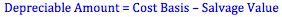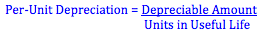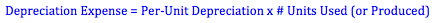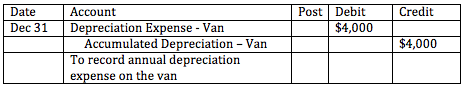Explore BrainMass
Share

# Units-of-Production Method of Depreciation

Units-of-activity depreciation (or units-of-production depreciation) is typically used in circumstances where the asset’s useful life is dependent on usage rather than the passage of time. For example, the useful life of a vehicle is often said to be in miles, not years. Under the activity approach, the depreciation expense for an asset will be higher in periods of high use and lower in periods of low use. In many circumstances, allocating expenses this way better matches expenses with the revenues they are associated with earning.

Activity methods of depreciation may be based on units of input (such as machine-hours) or units of output (such as the number of items it produces). Conceptually, the units of output produced is a better activity-measure than units of input; however, problems measuring output in many circumstances make input measures the more appropriate bases for computing depreciation.

Calculating Activity-Based Depreciation

Step 1: Calculate the depreciable amount. The depreciable amount is typically equal to the cost basis less the asset’s salvage value. The cost basis is the asset’s historical cost or book value. For example, if we purchase a van for \$20,000 and expect to sell it for \$4,000, we have a depreciable amount of \$16,000 (equal to \$20,000 - \$4,000).Step 2: Calculate the per-unit cost of usage. The per-unit cost of usage is calculated by dividing the depreciable amount by the number of activity units the asset is expected to use or produce in its useful life. For example, assume that we expect to drive our van for 160,000 miles in its useful life. We would have a per-unit cost of \$0.10/mile (equal to \$16,000 divided by 160,000 miles).Step 3: Calculate the depreciation expense for the period. To find the depreciation expense for the period we multiply the per-unit cost with the total usage of the machine during the period. For example, if we drive the van for 40,000 miles this year, we would have a depreciation expense of \$4,000 (equal to 40,000 miles multiplied by \$0.10/mile).Step 4: Record depreciation. To record depreciation, we debit an expense account for depreciation for the period and credit a contra-asset account typically called accumulated depreciation.When other methods of depreciation are used, the same accounts are debited and credited, but the amounts may be different.